Courses

# Frequency Domain Analysis Of Control Systems

## 10 Questions MCQ Test Mock Test Series for Electrical Engineering (EE) GATE 2020 | Frequency Domain Analysis Of Control Systems

Description
This mock test of Frequency Domain Analysis Of Control Systems for GATE helps you for every GATE entrance exam. This contains 10 Multiple Choice Questions for GATE Frequency Domain Analysis Of Control Systems (mcq) to study with solutions a complete question bank. The solved questions answers in this Frequency Domain Analysis Of Control Systems quiz give you a good mix of easy questions and tough questions. GATE students definitely take this Frequency Domain Analysis Of Control Systems exercise for a better result in the exam. You can find other Frequency Domain Analysis Of Control Systems extra questions, long questions & short questions for GATE on EduRev as well by searching above.
QUESTION: 1

Solution:
QUESTION: 2

Solution:
QUESTION: 3

### The initial slope of Bode plot for a transfer function having no poles at origin is

Solution:

With no-pole at origin, initial slope will be OdB/decade.
For n-pole at origin, initial slope will be -20 ndB/decade.

QUESTION: 4

The gain margin of a control system having the loop transfer function G(s)H(s) =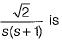Solution: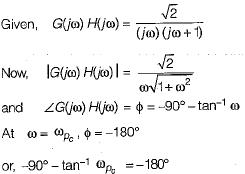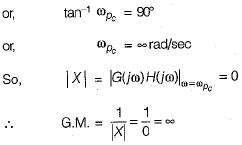QUESTION: 5

Gain crossover frequency is the frequency at which the gain of G(jω) is

Solution: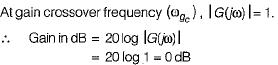QUESTION: 6

For a control system having gain margin of -10 dB, the magnitude of GH(s) for 180° phase shift is

Solution: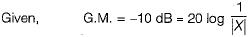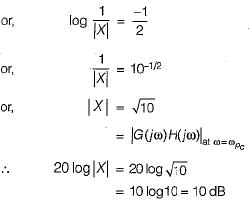QUESTION: 7

If the Nyquist plot of the loop transfer function G(s)H(s) of a closed-loop system does not encloses the (-1, j0) point in the G(s)H(s) plane, the gain margin of the system will be

Solution:

Since the critical point (-1 + j0 ) is not enclosed, therefore m agnitude of OLTF,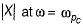will be less than unity.
Therefore, G.M. = 20 log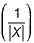will be positive and the system will be stable.

QUESTION: 8

A system with phase margin close to zero or gain margin close to unity is

Solution:
QUESTION: 9

If the transfer function of a first-order system in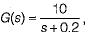then the time constant of this system will be

Solution: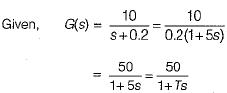Hence, time constant = T= 5 seconds

QUESTION: 10

The polar plot of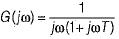Solution: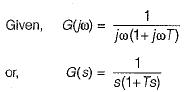Given system is of type-1 and order-2, therefore its polar plot will be as shown below.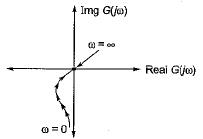Hence, the polar plot will neither cross the real axis nor the imaginary axis.